KINEMATICS (MOTION SPEED VELOCITY) CHEAT SHEET

Distance: Distance is a scalar quantity representing the interval between two points. It is just the magnitude of the interval.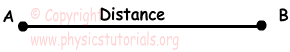Displacement: Displacement can be defined as distance between the initial and final point of an object. It is a vector quantity having both magnitude and direction.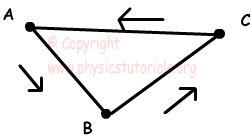Displacement is zero

Distance taken is=lABl+lBCl+lCAl

Speed: Speed can be defined as “how fast something moves” or it can be explained more scientifically as “the distance covered in a unit of time”. Speed is a scalar quantity.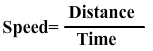Velocity: It can be defined as “speed having direction” or displacement in a unit of time. Velocity is a vector quantity and it has both magnitude and direction.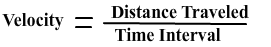Acceleration: We can easily define acceleration as “change in velocity”. This change can be in the magnitude (speed) of the velocity or in the direction of the velocity.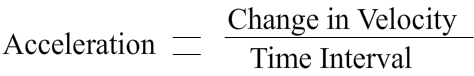Equations of Linear Motion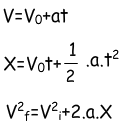Free Fall: Motion of an object dropped from a height without initial velocity. Only force acting on an object is its weight or gravitational force. Equations of free fall;

V=g.t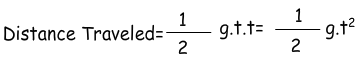Kinematics Exams and Solutions

Related